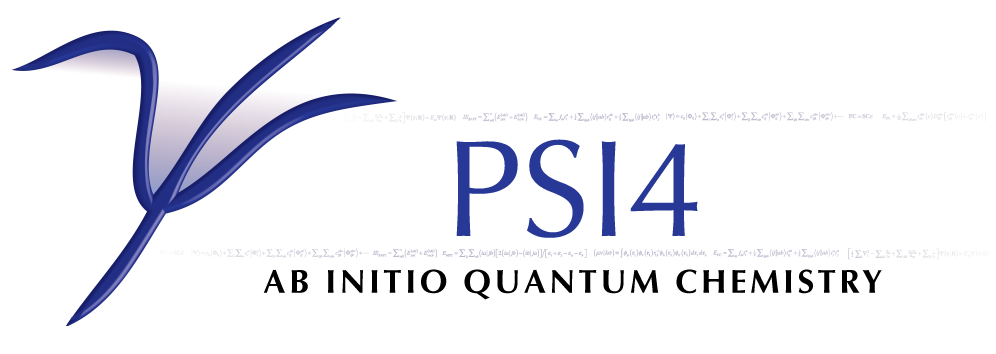# DCFT¶

Performs Density Cumulant Functional Theory computations

## General¶

### ALGORITHM¶

The algorithm to use for the density cumulant and orbital updates in the energy computation. Two-step algorithm (default) is generally more efficient and shows better convergence than simultaneous

• Type: string
• Possible Values: TWOSTEP, SIMULTANEOUS
• Default: TWOSTEP

### AO_BASIS¶

Controls whether to avoid the AO->MO transformation of the two-electron integrals for the four-virtual case (<VV||VV>) by computing the corresponding terms in the AO basis. AO_BASIS = DISK algorithm reduces the memory requirements. It is, however, less efficient due to the extra I/O, so the default algorithm is preferred.

• Type: string
• Possible Values: NONE, DISK
• Default: NONE

### CACHELEVEL¶

Controls how to cache quantities within the DPD library

• Type: integer
• Default: 2

### DAMPING_PERCENTAGE¶

The amount (percentage) of damping to apply to the orbital update procedure: 0 will result in a full update, 100 will completely stall the update. A value around 20 (which corresponds to 20% of the previous iteration’s density being mixed into the current iteration) can help in cases where oscillatory convergence is observed.

• Type: double
• Default: 0.0

### DIIS_MAX_VECS¶

Maximum number of error vectors stored for DIIS extrapolation

• Type: integer
• Default: 6

### DIIS_MIN_VECS¶

Minimum number of error vectors stored for DIIS extrapolation

• Type: integer
• Default: 3

### DIIS_START_CONVERGENCE¶

Value of RMS of the density cumulant residual and SCF error vector below which DIIS extrapolation starts. Same keyword controls the DIIS extrapolation for the solution of the response equations.

### IGNORE_TAU¶

Controls whether to ignore terms containing non-idempotent contribution to OPDM or not (for debug puproses only). For practical applications only the default must be used

### INTS_TOLERANCE¶

Minimum absolute value below which integrals are neglected

### LAMBDA_MAXITER¶

Maximum number of density cumulant update micro-iterations per macro-iteration (for ALOGRITHM = TWOSTEP). Same keyword controls the maximum number of density cumulant response micro-iterations per macro-iteration for the solution of the response equations (for RESPONSE_ALOGRITHM = TWOSTEP)

• Type: integer
• Default: 50

### LOCK_OCC¶

Controls whether to force the occupation to be that of the SCF guess. For practical applications only the default must be used

### MAXITER¶

Maximum number of macro-iterations for both energy and the solution of the response equations

• Type: integer
• Default: 40

### MO_RELAX¶

Controls whether to relax the orbitals during the energy computation or not (for debug puproses only). For practical applications only the default must be used

### RESPONSE_ALGORITHM¶

The algorithm to use for the solution of the response equations for the analytic gradients and properties. Two-step algorithm is generally more efficient than simultaneous and is used by default

• Type: string
• Possible Values: TWOSTEP, SIMULTANEOUS
• Default: TWOSTEP

### R_CONVERGENCE¶

Convergence criterion for the RMS of the residual vector in the density cumulant updates as well as the solution of the density cumulant and orbital response equations. In the orbital updates controls the RMS of the SCF error vector

### SCF_MAXITER¶

Maximum number of orbital update micro-iterations per macro-iteration (for ALOGRITHM = TWOSTEP). Same keyword controls the maximum number of orbital response micro-iterations per macro-iteration for the solution of the response equations (for RESPONSE_ALOGRITHM = TWOSTEP)

• Type: integer
• Default: 50

### TAU_SQUARED¶

Controls whether to compute the DCFT energy with the Tau^2 correction to Tau

### TIKHONOW_OMEGA¶

The shift applied to the denominator in the density cumulant update iterations

• Type: double
• Default: 0.0

### TPDM¶

Controls whether to compute unrelaxed two-particle density matrix at the end of the energy computation